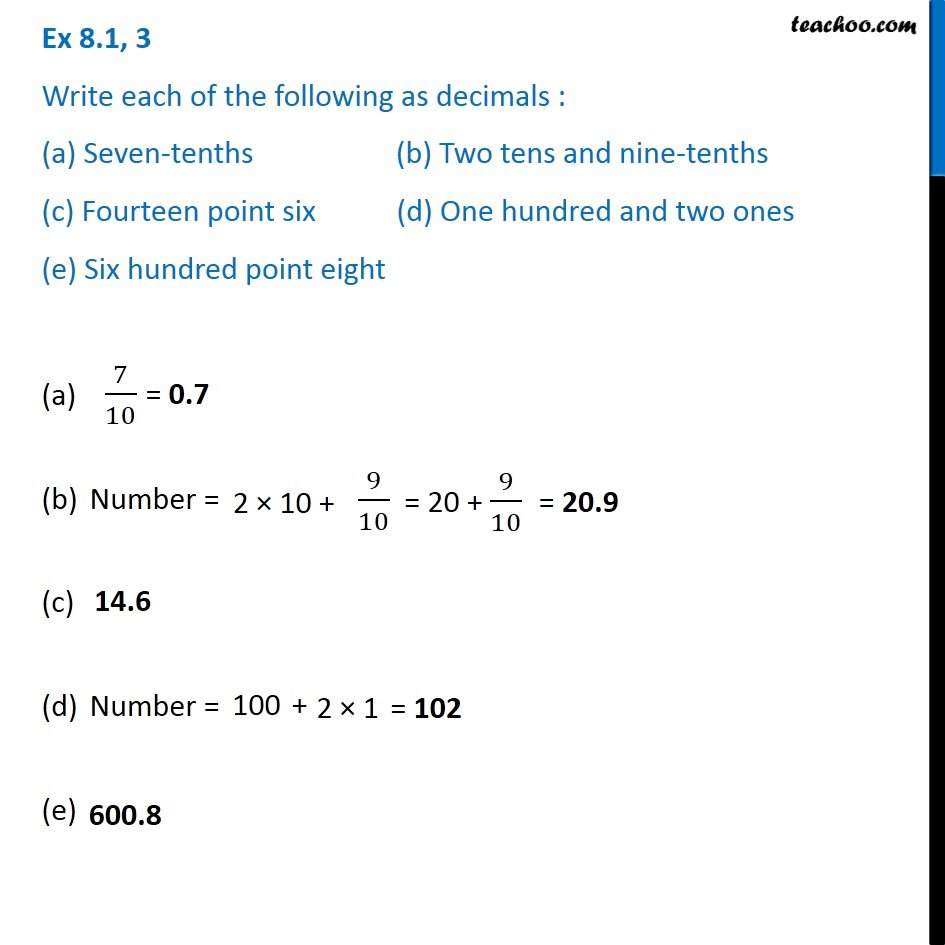Ex 8.1

Chapter 8 Class 6 Decimals
Serial order wiseGet live Maths 1-on-1 Classs - Class 6 to 12

### Transcript

Ex 8.1, 3 Write each of the following as decimals : (a) Seven-tenths (b) Two tens and nine-tenths (c) Fourteen point six (d) One hundred and two ones (e) Six hundred point eight Number = Number = (e)